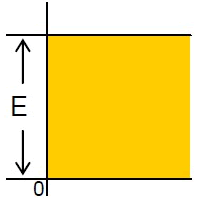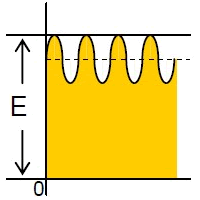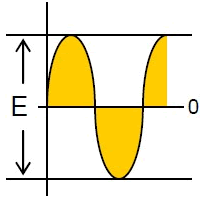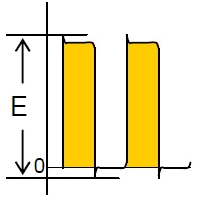# Applied voltage

Safety Application Guide for Multilayer Ceramic Chip Capacitors
Design
Electrical factors
Applied voltage

#### Voltage which is applied to the capacitor should not exceed the rated voltage given in the specifications.

1. (1) Applying overvoltage to a capacitor may cause dielectric breakdown and result in a short circuit.
The duration until dielectric breakdown depends on the applied voltage and the ambient temperature.
2. (2) When pulse voltage with a very short rising time or AC voltage of a high frequency is applied to capacitors, even though the voltage is less than or
equal to the rated voltage, the reliability of the capacitor may be influenced. (See Applied voltage and self-heating temperature)
3. (3) When AC voltage is superimposed on DC voltage, the zero-to-peak voltage shall not exceed the rated
voltage.When AC voltage or pulse voltage is applied, the peak-to-peak voltage shall not exceed the rated voltage.

DC voltage
DC + AC voltage
AC voltage
Pulse voltageNOTE Maximum possibly applied voltage.

4. (4) Abnormal voltage (surge voltage, static electricity, pulse voltage, etc.) shall not exceed the rated voltage.

When capacitors are used in a series connection, it is necessary to add a balancing circuit such as voltage dividing resistors in order to avoid an
imbalance in the voltage applied to each capacitor.

Concerning overvoltage on AC capacitors (Electromagnetic interference suppression capacitors)
These capacitors shall be selected in consideration of the possibility that the supply voltage of the
system may rise by up to 10 % of its nominal voltage.